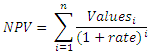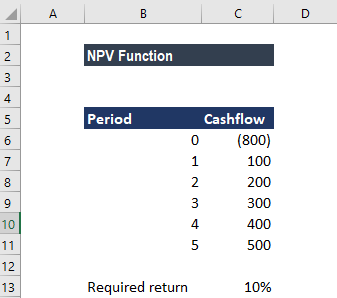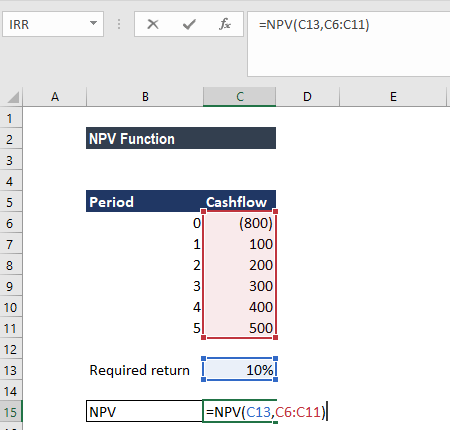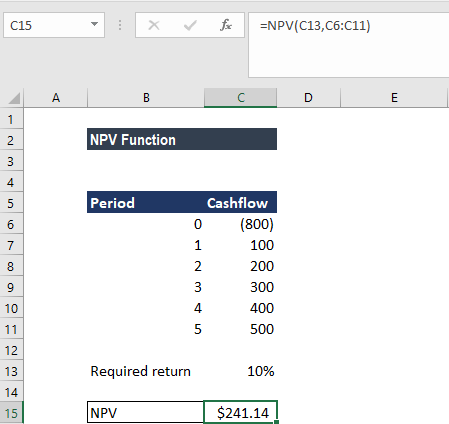# NPV Function

Get the Net Present Value (NPV) for periodic cash flows

## What is the NPV Function?

The NPV Function is an Excel Financial function that will calculate the Net Present Value (NPV) for a series of cash flows and a given discount rate. It is important to understand the Time Value of Money, which is a foundational building block of various Financial Valuation methods.

In financial modeling, the NPV function is useful in determining the value of an investment or understanding the feasibility of a project.  It should be noted that it’s usually more appropriate for analysts to use the XNPV function instead of the regular NPV function.

### Formula

=NPV(rate,value1,[value2],…)

The NPV function uses the following arguments:

1. Rate (required argument) – This is the rate of discount over the length of the period.
2. Value1, Value2 – Value1 is a required option. They are numeric values that represent a series of payments and income where:
• Negative payments represent outgoing payments.
• Positive payments represent incoming payments.

The NPV function uses the following equation to calculate the Net Present Value of an Investment:### How to use the NPV Function in Excel?

To understand the uses of the function, let’s consider a few examples:

#### Example – Using the Function

Suppose we are given the following data on cash inflows and outflows:The required rate of return is 10%. To calculate the NPV, we will use the formula below:We get the result below:The NPV formula is based on future cash flows. If the first cash flow occurs at the start of the first period, the first value must be added to the NPV result, not included in the values arguments.

### Things to remember

1. Arguments must be numerical, or function with a numerical output. Other forms of input will result in an error.
2. Arrays that are used as input will only have its numerical values evaluated. All other values in the array will be ignored.
3. The input order matters for the series of cash flows.
4. The NPV function assumes that payments are spaced on equal periodic payments.
5. The NPV function and IRR function (Internal Rate of Return) are closely related. IRR is the rate for which the NPV equals zero.

Thanks for reading CFI’s guide to important Excel functions! By taking the time to learn and master these functions, you’ll significantly speed up your financial analysis. To learn more, check out these additional CFI resources:

• Excel Functions for Finance